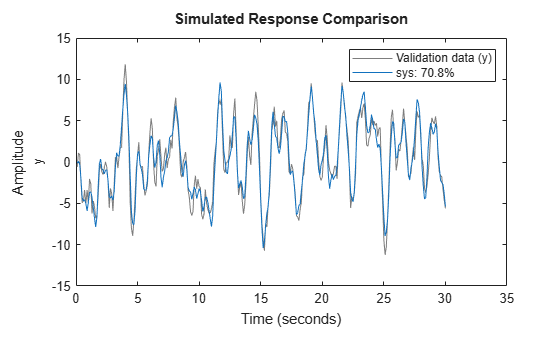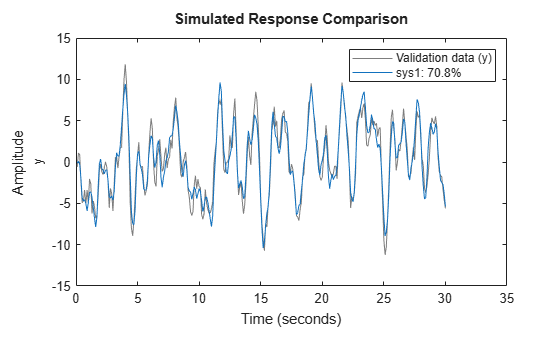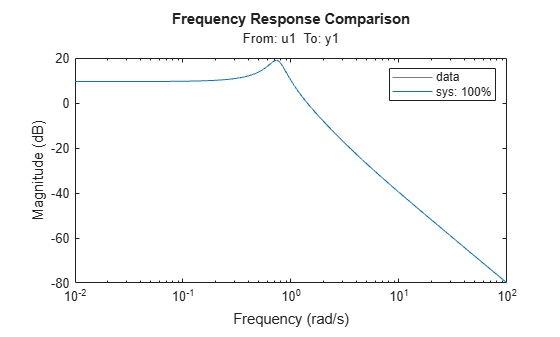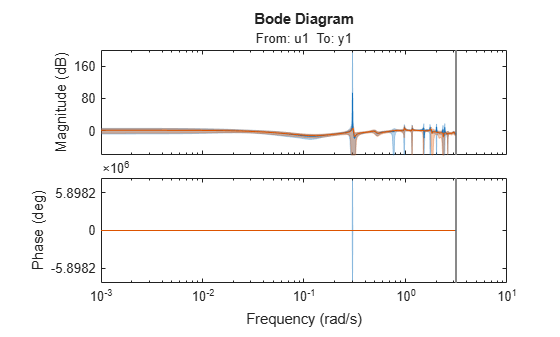Main Content

# oe

Estimate output-error polynomial model using time-domain or frequency-domain data

## Syntax

``````sys = oe(data,[nb nf nk])``````
``````sys = oe(data,[nb nf nk],Name,Value)``````
``sys = oe(data,init_sys)``
``sys = oe(data,___,opt)``
``[sys,ic] = oe(___)``

## Description

Output-error (OE) models are a special configuration of polynomial models, having only two active polynomials—B and F. OE models represent conventional transfer functions that relate measured inputs to outputs while also including white noise as an additive output disturbance. You can estimate OE models using time- and frequency-domain data. The `tfest` command offers the same functionality as `oe`. For `tfest`, you specify the model orders using number of poles and zeros rather than polynomial degrees. For continuous-time estimation, `tfest` provides faster and more accurate results, and is recommended.

example

``````sys = oe(data,[nb nf nk])``` estimates an OE model `sys`, represented by$y\left(t\right)=\frac{B\left(q\right)}{F\left(q\right)}u\left(t-nk\right)+e\left(t\right)$y(t) is the output, u(t) is the input, and e(t) is the error.`oe` estimates `sys` using the measured input-output data `data`, which can be in the time or the frequency domain. The orders `[nb nf nk]` define the number of parameters in each component of the estimated polynomial.```

example

``````sys = oe(data,[nb nf nk],Name,Value)``` specifies model structure attributes using additional options specified by one or more name-value pair arguments.```
````sys = oe(data,init_sys)` uses the linear system `init_sys` to configure the initial parameterization of `sys`.```

example

````sys = oe(data,___,opt)` estimates a polynomial model using the option set `opt` to specify estimation behavior. You can use this syntax with any of the previous input-argument combinations.```

example

````[sys,ic] = oe(___)` returns the estimated initial conditions as an `initialCondition` object. Use this syntax if you plan to simulate or predict the model response using the same estimation input data and then compare the response with the same estimation output data. Incorporating the initial conditions yields a better match during the first part of the simulation.```

## Examples

collapse all

Estimate an OE polynomial from time-domain data using two methods to specify input delay.

Load the estimation data.

`load iddata1 z1`

Set the orders of the B and F polynomials `nb` and `nf`. Set the input delay `nk` to one sample. Compute the model `sys`.

```nb = 2; nf = 2; nk = 1; sys = oe(z1,[nb nf nk]);```

Compare the simulated model response with the measured output.

`compare(z1,sys)`The plot shows that the fit percentage between the simulated model and the estimation data is greater than 70%.

Instead of using `nk`, you can also use the name-value pair argument `'InputDelay'` to specify the one-sample delay.

```nk = 0; sys1 = oe(z1,[nb nf nk],'InputDelay',1); figure compare(z1,sys1)```The results are identical.

You can view more information about the estimation by exploring the `idpoly` property `sys.Report`.

`sys.Report`
```ans = Status: 'Estimated using OE' Method: 'OE' InitialCondition: 'zero' Fit: [1x1 struct] Parameters: [1x1 struct] OptionsUsed: [1x1 idoptions.polyest] RandState: [1x1 struct] DataUsed: [1x1 struct] Termination: [1x1 struct] ```

For example, find out more information about the termination conditions.

`sys.Report.Termination`
```ans = struct with fields: WhyStop: 'Near (local) minimum, (norm(g) < tol).' Iterations: 3 FirstOrderOptimality: 0.0708 FcnCount: 7 UpdateNorm: 1.4809e-05 LastImprovement: 5.1744e-06 ```

The report includes information on the number of iterations and the reason the estimation stopped iterating.

Load the estimation data.

`load oe_data1 data;`

`The idfrd` object `data` contains the continuous-time frequency response for the following model:

`$G\left(s\right)=\frac{s+3}{{s}^{3}+2{s}^{2}+s+1}$`

Estimate the model.

```nb = 2; nf = 3; sys = oe(data,[nb nf]);```

Evaluate the goodness of fit.

`compare(data,sys);`Estimate a high-order OE model from data collected by simulating a high-order system. Determine the regularization constants by trial and error and use the values for model estimation.

Load the data.

`load regularizationExampleData.mat m0simdata`

Estimate an unregularized OE model of order 30.

`m1 = oe(m0simdata,[30 30 1]);`

Obtain a regularized OE model by determining the Lambda value using trial and error.

```opt = oeOptions; opt.Regularization.Lambda = 1; m2 = oe(m0simdata,[30 30 1],opt);```

Compare the model outputs with the estimation data.

```opt = compareOptions('InitialCondition','z'); compare(m0simdata,m1,m2,opt);```The regularized model `m2 `produces a better fit than the unregularized model `m1`.

Compare the variance in the model responses.

```h = bodeplot(m1,m2); opt = getoptions(h); opt.PhaseMatching = 'on'; opt.ConfidenceRegionNumberSD = 3; opt.PhaseMatching = 'on'; setoptions(h,opt); showConfidence(h);```The regularized model `m2` has a reduced variance compared to the unregularized model `m1`.

Load the estimation data `data` and sample time `Ts`.

`load oe_data2.mat data Ts`

An `iddata` object `data` contains the discrete-time frequency response for the following model:

`$G\left(s\right)=\frac{1000}{s+500}$`

View the estimation sample time `Ts` that you loaded.

`Ts`
```Ts = 1.0000e-03 ```

This value matches the property `data.Ts`.

`data.Ts`
```ans = 1.0000e-03 ```

You can estimate a continuous model from `data` by limiting the input and output frequency bands to the Nyquist frequency. To do so, specify the estimation prefilter option `'WeightingFilter`' to define a passband from `0` to `0.5*pi/Ts` rad/s. The software ignores any response values with frequencies outside of that passband.

`opt = oeOptions('WeightingFilter',[0 0.5*pi/Ts]);`

Set the `Ts` property to `0` to treat `data` as continuous-time data.

`data.Ts = 0;`

Estimate the continuous model.

```nb = 1; nf = 3; sys = oe(data,[nb nf],opt);```

Load the data.

`load iddata1ic z1i`

Estimate an OE polynomial model `sys` and return the initial conditions in `ic`.

```nb = 2; nf = 2; nk = 1; [sys,ic] = oe(z1i,[nb,nf,nk]); ic```
```ic = initialCondition with properties: A: [2x2 double] X0: [2x1 double] C: [0.9428 0.4824] Ts: 0.1000 ```

`ic` is an `initialCondition` object that encapsulates the free response of `sys`, in state-space form, to the initial state vector in `X0`. You can incorporate `ic` when you simulate `sys` with the `z1i` input signal and compare the response with the `z1i` output signal.

## Input Arguments

collapse all

Estimation data, specified as an `iddata` object, an `frd` object, or an `idfrd` object.

For time-domain estimation, `data` must be an `iddata` object containing the input and output signal values.

For frequency-domain estimation, `data` can be one of the following:

• Recorded frequency response data (`frd` (Control System Toolbox) or `idfrd`)

• `iddata` object with properties specified as follows:

• `InputData` — Fourier transform of the input signal

• `OutputData` — Fourier transform of the output signal

• `Domain``'Frequency'`

Time-domain estimation data must be uniformly sampled. By default, the software sets the sample time of the model to the sample time of the estimation data.

For multiexperiment data, the sample times and intersample behavior of all the experiments must match.

You can compute discrete-time models from time-domain data or discrete-time frequency-domain data. Use `tfest` to compute continuous-time models.

OE model orders, specified as a 1-by-3 vector or a vector of integer matrices.

For a system represented by

`$y\left(t\right)=\frac{B\left(q\right)}{F\left(q\right)}u\left(t-nk\right)+e\left(t\right)$`

where y(t) is the output, u(t) is the input, and e(t) is the error, the elements of ```[nb nf nk]``` are as follows:

• `nb` — Order of the B(q) polynomial + 1, which is equivalent to the length of the B(q) polynomial. `nb` is an Ny-by-Nu matrix. Ny is the number of outputs and Nu is the number of inputs.

• `nf` — Order of the F polynomial. `nf` is an Ny-by-Nu matrix.

• `nk` — Input delay, expressed as the number of samples. `nk` is an Ny-by-Nu matrix. The delay appears as leading zeros of the B polynomial.

For estimation using continuous-time frequency-domain data, specify only `[nb nf]` and omit `nk`. For an example, see Estimate Continuous-Time OE Model Using Frequency Response.

Linear system that configures the initial parameterization of `sys`, specified as an `idpoly` model, another linear model, or a structure. You obtain `init_sys` either by performing an estimation using measured data or by direct construction.

If `init_sys` is an `idpoly` model of the OE structure, `oe` uses the parameter values of `init_sys` as the initial guess for estimating `sys`. The sample time of `init_sys` must match the sample time of the data.

Use the `Structure` property of `init_sys` to configure initial guesses and constraints for B(q) and F(q). For example:

• To specify an initial guess for the F(q) term of `init_sys`, set `init_sys.Structure.F.Value` as the initial guess.

• To specify constraints for the B(q) term of `init_sys`:

• Set `init_sys.Structure.B.Minimum` to the minimum B(q) coefficient values.

• Set `init_sys.Structure.B.Maximum` to the maximum B(q) coefficient values.

• Set `init_sys.Structure.B.Free` to indicate which B(q) coefficients are free for estimation.

If `init_sys` is not a polynomial model of the OE structure, the software first converts `init_sys` to an OE structure model. `oe` uses the parameters of the resulting model as the initial guess for estimating `sys`.

If you do not specify `opt` and `init_sys` was obtained by estimation, then the software uses estimation options from `init_sys.Report.OptionsUsed`.

Estimation options, specified as an `oeOptions` option set. Options specified by `opt` include:

• Estimation objective

• Handling of initial conditions

• Numerical search method and the associated options

For examples of specifying estimation options, see Estimate Continuous Model Using Band-Limited Discrete-Time Frequency-Domain Data.

### Name-Value Pair Arguments

Specify optional comma-separated pairs of `Name,Value` arguments. `Name` is the argument name and `Value` is the corresponding value. `Name` must appear inside quotes. You can specify several name and value pair arguments in any order as `Name1,Value1,...,NameN,ValueN`.

Example: `'InputDelay',1`

Input delays for each input channel, specified as the comma-separated pair consisting of `'InputDelay'` and a numeric vector.

• For continuous-time models, specify `'InputDelay'` in the time units stored in the `TimeUnit` property.

• For discrete-time models, specify `'InputDelay'` in integer multiples of the sample time `Ts`. For example, setting `'InputDelay'` to `3` specifies a delay of three sampling periods.

For a system with Nu inputs, set `InputDelay` to an Nu-by-1 vector. Each entry of this vector is a numerical value that represents the input delay for the corresponding input channel.

To apply the same delay to all channels, specify `'InputDelay'` as a scalar.

For an example, see Estimate OE Polynomial Model.

Transport delays for each input-output pair, specified as the comma-separated pair consisting of `'IODelay'` and a numeric array.

• For continuous-time models, specify `'IODelay'` in the time units stored in the `TimeUnit` property.

• For discrete-time models, specify `'IODelay'` in integer multiples of the sample time `Ts`. For example, setting `'IODelay'` to `4` specifies a transport delay of four sampling periods.

For a system with Nu inputs and Ny outputs, set `'IODelay'` to an Ny-by-Nu matrix. Each entry is an integer value representing the transport delay for the corresponding input-output pair.

To apply the same delay to all channels, specify `'IODelay'` as a scalar.

You can specify `'IODelay'` as an alternative to the `nk` value. Doing so simplifies the model structure by reducing the number of leading zeros in the B polynomial. In particular, you can represent `max(nk-1,0)` leading zeros as input-output delays using `'IODelay'` instead.

## Output Arguments

collapse all

OE polynomial model that fits the estimation data, returned as an `idpoly` model object. This model is created using the specified model orders, delays, and estimation options. The sample time of `sys` matches the sample time of the estimation data. Therefore, `sys` is always a discrete-time model when estimated from time-domain data. For continuous-time model identification using time-domain data, use `tfest`.

The `Report` property of the model stores information about the estimation results and options used. `Report` has the following fields.

Report FieldDescription
`Status`

Summary of the model status, which indicates whether the model was created by construction or obtained by estimation.

`Method`

Estimation command used.

`InitialCondition`

Handling of initial conditions during model estimation, returned as one of the following values:

• `'zero'` — The initial conditions were set to zero.

• `'estimate'` — The initial conditions were treated as independent estimation parameters.

• `'backcast'` — The initial conditions were estimated using the best least squares fit.

This field is especially useful to view how the initial conditions were handled when the `InitialCondition` option in the estimation option set is `'auto'`.

`Fit`

Quantitative assessment of the estimation, returned as a structure. See Loss Function and Model Quality Metrics for more information on these quality metrics. The structure has the following fields:

FieldDescription
`FitPercent`

Normalized root mean squared error (NRMSE) measure of how well the response of the model fits the estimation data, expressed as the percentage `fit` = 100(1-NRMSE).

`LossFcn`

Value of the loss function when the estimation completes.

`MSE`

Mean squared error (MSE) measure of how well the response of the model fits the estimation data.

`FPE`

Final prediction error for the model.

`AIC`

Raw Akaike Information Criteria (AIC) measure of model quality.

`AICc`

Small sample-size corrected AIC.

`nAIC`

Normalized AIC.

`BIC`

Bayesian Information Criteria (BIC).

`Parameters`

Estimated values of model parameters.

`OptionsUsed`

Option set used for estimation. If no custom options were configured, this is a set of default options. See `oeOptions` for more information.

`RandState`

State of the random number stream at the start of estimation. Empty, `[]`, if randomization was not used during estimation. For more information, see `rng`.

`DataUsed`

Attributes of the data used for estimation, returned as a structure with the following fields:

FieldDescription
`Name`

Name of the data set.

`Type`

Data type.

`Length`

Number of data samples.

`Ts`

Sample time.

`InterSample`

Input intersample behavior, returned as one of the following values:

• `'zoh'` — Zero-order hold maintains a piecewise-constant input signal between samples.

• `'foh'` — First-order hold maintains a piecewise-linear input signal between samples.

• `'bl'` — Band-limited behavior specifies that the continuous-time input signal has zero power above the Nyquist frequency.

`InputOffset`

Offset removed from time-domain input data during estimation. For nonlinear models, it is `[]`.

`OutputOffset`

Offset removed from time-domain output data during estimation. For nonlinear models, it is `[]`.

`Termination`

Termination conditions for the iterative search used for prediction error minimization, returned as a structure with the following fields:

FieldDescription
`WhyStop`

Reason for terminating the numerical search.

`Iterations`

Number of search iterations performed by the estimation algorithm.

`FirstOrderOptimality`

$\infty$-norm of the gradient search vector when the search algorithm terminates.

`FcnCount`

Number of times the objective function was called.

`UpdateNorm`

Norm of the gradient search vector in the last iteration. Omitted when the search method is `'lsqnonlin'` or `'fmincon'`.

`LastImprovement`

Criterion improvement in the last iteration, expressed as a percentage. Omitted when the search method is `'lsqnonlin'` or `'fmincon'`.

`Algorithm`

Algorithm used by `'lsqnonlin'` or `'fmincon'` search method. Omitted when other search methods are used.

For estimation methods that do not require numerical search optimization, the `Termination` field is omitted.

For more information on using `Report`, see Estimation Report.

Estimated initial conditions, returned as an `initialCondition` object or an object array of `initialCondition` values.

• For a single-experiment data set, `ic` represents, in state-space form, the free response of the transfer function model (A and C matrices) to the estimated initial states (x0).

• For a multiple-experiment data set with Ne experiments, `ic` is an object array of length Ne that contains one set of `initialCondition` values for each experiment.

If `oe` returns `ic` values of `0` and the you know that you have non-zero initial conditions, set the `'InitialCondition'` option in `oeOptions` to `'estimate'` and pass the updated option set to `oe`. For example:

```opt = oeOptions('InitialCondition,'estimate') [sys,ic] = oe(data,np,nz,opt)```
The default `'auto'` setting of `'InitialCondition'` uses the `'zero'` method when the initial conditions have a negligible effect on the overall estimation-error minimization process. Specifying `'estimate'` ensures that the software estimates values for `ic`.

For more information, see `initialCondition`. For an example of using this argument, see Obtain Initial Conditions.

## More About

collapse all

### Output-Error (OE) Model

The general output-error model structure is:

`$y\left(t\right)=\frac{B\left(q\right)}{F\left(q\right)}u\left(t-{n}_{k}\right)+e\left(t\right)$`

The orders of the output-error model are:

### Continuous-Time Output-Error Model

If `data` is continuous-time frequency-domain data, `oe` estimates a continuous-time model with the following transfer function:

`$G\left(s\right)=\frac{B\left(s\right)}{F\left(s\right)}=\frac{{b}_{nb}{s}^{\left(nb-1\right)}+{b}_{nb-1}{s}^{\left(nb-2\right)}+...+{b}_{1}}{{s}^{nf}+{f}_{nf}{s}^{\left(nf-1\right)}+...+{f}_{1}}$`

The orders of the numerator and denominator are `nb` and `nf`, similar to the discrete-time case. However, the sample delay `nk` does not exist in the continuous case, and you should not specify `nk` when you command the estimation. Instead, express any system delay using the name-value pair argument `'IODelay'` along with the system delay in the time units that are stored in the property `TimeUnit`. For example, suppose that your continuous system has a delay of `iod` seconds. Use `model = oe(data,[nb nf],'IODelay',iod)`.

## Extended Capabilities

Introduced before R2006a

## Support평가판 신청